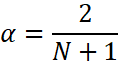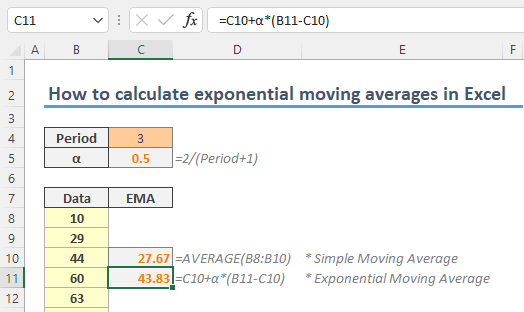Exponential moving average option is a data smoothing approach which gives exponentially decreasing weights to values over time. In this guide, we’re going to show you how to calculate exponential moving average in Excel.

## What is exponential moving average (EMA)?

Exponential moving average (EMA), also known as exponentially weighted moving average (EWMA) is form of weighted moving average which assumes that more current data points are more important since they are more relevant than older data points. The difference is that the weights are distributed exponentially.The formula of EMA is the following where,

• the coefficient α a constant smoothing factor between 0 and 1 and
• n is the period.You can use simple moving average when calculating the first EMA (n), since there will not be a previous time (n-1).

If you are interested in Simple Moving Average (SMA), check out How to calculate simple moving average in Excel article.

You can calculate the α easily by the following formula. The N represents the number of data values in a period.Let's use these formulas on an example to calculate exponential moving average in Excel.

## Calculating exponential moving average

The first step is to find the α value. Simply apply the formula into your worksheet. In our example, we are calculating a three-point EMA, which is defined in cell C4. Thus, the α value for three-points is 0.5, 2 / (3 + 1).Since our period includes three points, we need the average of the first 3 values. Use the AVERAGE formula to find it.Finally, we can use the EMA formula starting from the 4th data point.

Previous EMA (n-1): C10

Price (n): B11Next step is to copy the formula down along with data. The important point is to avoid the \$ signs in the range reference to keep the range relative while keeping weight range absolute. Excel adjusts relative references based on the formula cell's location. Thus, you do not need to update your formula each time.

You can learn about relative reference in How to create an Excel absolute reference and relative reference.